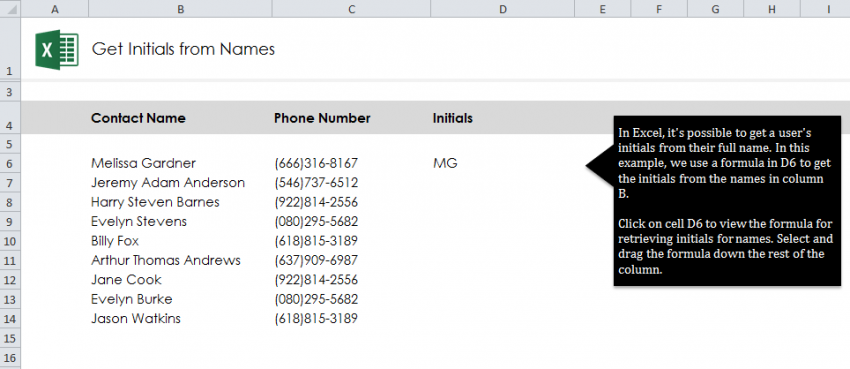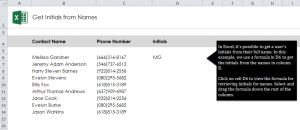# Retrieve Initials from Name in Excel13
AprLearn how to make initials in Excel spreadsheets from someone’s full name. You can use a formula to retrieve initials from first, middle, and last names in a contacts list. This is a great formula to know how to use in Excel if you are organizing a large number of names. You can search alphabetically through the initials or export the data to use as an employee list, a check list, or whatever you need initials for.

Download the example spreadsheet to follow along and learn the formula for creating initials in Excel.

Open up your spreadsheet example and you will see a list of contacts in one column, and another column where you will create initials. The first contact has already had the initials added to show you how it will look. To try to the formula yourself, click on cell D7 and enter this formula:

=IF(LEN(B7)-LEN(SUBSTITUTE(B7,” “,””))=0,LEFT(B7,1),IF(LEN(B7)-LEN(SUBSTITUTE(B7,” “,””))=1,LEFT(B7,1)&MID(B7,FIND(” “,B7)+1,1),LEFT(B7,1)&MID(B7,FIND(” “,B7)+1,1)&MID(B7,FIND(” “,B7,FIND(” “,B7)+1)+1,1)))

Hit enter, and D7 should now read “JAA”.

You can now apply this formula to all of the names on the contact list by dragging the corner of D7 down the D column to D14. This will apply the formula to each name on the list so you don’t have to type out the formula for each entry.

In your own contacts sheet, you can take the above formula and change each entry of “B7” to the correct cell with the name of the contact you want to retrieve initials from. Then you can drag that cell where you want the formula applied and your contacts list will be finished.